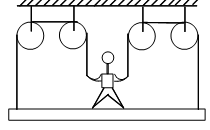A painter of mass M stands on a platform of mass m and pulls himself up by two ropes which            hang over pulley as shown. He pulls each rope with force F and moves upwards with a uniform            acceleration ‘a’ find ‘a’ neglecting the fact that no one could do this for long time

# A painter of mass M stands on a platform of mass m and pulls himself up by two ropes which            hang over pulley as shown. He pulls each rope with force F and moves upwards with a uniform            acceleration ‘a’ find ‘a’ neglecting the fact that no one could do this for long time1. A

$\frac{4\text{F}}{\text{M}+\text{m}}-\text{g}$

2. B

$\frac{3\text{F}}{\text{M}+\text{m}}-\text{g}$

3. C

$\frac{4\text{F}}{\text{M}-\text{m}}+\text{g}$

4. D

$\frac{3\text{F}}{\text{M}-\text{m}}+\text{g}$

Register to Get Free Mock Test and Study Material

+91

Verify OTP Code (required)